# Texas Go Math Grade 8 Lesson 16.4 Answer Key Estimating College Costs and Payments

Refer to our Texas Go Math Grade 8 Answer Key Pdf to score good marks in the exams. Test yourself by practicing the problems from Texas Go Math Grade 8 Lesson 16.4 Answer Key Estimating College Costs and Payments.

## Texas Go Math Grade 8 Lesson 16.4 Answer Key Estimating College Costs and Payments

Example 1

June wants to attend Texas A&M University-Kingsville, near Corpus Christi, Texas. She is 18, single, does not have any dependents, and lives in Dallas. She was raised by her single father, a contractor who makes $81,000 per year and pays roughly 12% income tax. For the past 4 years, June has worked part time at the local bookstore, earning a taxable annual income of$15,000, which is taxed at roughly 8%. June has 2 brothers, both of whom are in middle school.

How much should June expect to spend if she plans on completing a four-year degree program at A&M University-Kingsville while living in on-campus housing?
STEP 1: Find the cost of attending Texas A&M University-Kingsville for 1 year using the values in the table.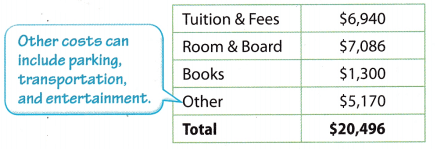STEP 2: Compute the cost of attending the university for 4 years.
$20,496 × 4 =$81,984
The estimated cost of June attending for 4 years is $81,984. Reflect Question 1. How can June help to pay for her education? Answer: June has worked part time at the local bookstore, earning a taxable annual income of$15,000. It is taxed at roughly 8%.

Question 2.
June is also considering attending Del Mar College in Corpus Christi to get a 2-year associate’s degree. Estimate the cost of June attending Del Mar College. Use the college’s website or another online tool to find the figures for an out-of-district student.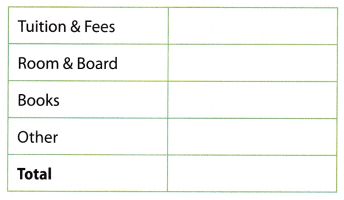The total amount of June tuition and fees at the Del Mar College for one year = is $5738. The total amount of June tuition and fees at the Del Mar College for two years =$5738 × 2 = $11,476 The total amount of June room and board fee for one year = is$6013.
The total amount of June room and board fee for two years = $6013 × 2 =$12,026
The total amount for June books for one year = $3820. The total amount for June books for two year =$3820 × 2 = $7640 The total amount for June and other purposes for one year =$1000.
The total amount for June and other purposes for two years = $1000 × 2 =$2000
The total cost of June attending Del Mar College for two years = $11,476 +$12,026 + $7640 +$2000 = $33,142. Question 3. Suppose June earns an associate’s degree from Del Mar and then transfers to Texas A&M University-Kingsville for two more years to complete a bachelor’s degree. Estimate the total amount that the 4 years of school will cost. Answer: The total amount of June tuition and fees at the Del Mar College for one year =$5738.
The total amount of June tuition and fees at the Del Mar College for two years = $5738 × 2 =$11,476
The total amount of June room and board fee for one year = $6013. The total amount of June room and board fee for two years =$6013 × 2 = $12,026 The total amount for June books for one year =$3820.
The total amount for June books for two year = $3820 × 2 =$7640
The total amount for June and other purposes for one year = $1000. The total amount for June and other purposes for two years =$1000 × 2 = $2000. The total cost of June attending Del Mar College for two years =$11,476 + $12,026 +$7640 + $2000 =$33,142.
June wants to attend Texas A&M University-Kingsville college for one year = $20,494. June wants to attend Texas A&M University-Kingsville college for two years =$20,494 × 2 = 40,988
The total amount that the 4 years of school will cost = $33,142 +$40,988 = $74,130 Question 4. Approximately how much less would it cost June to attend Del Mar for two years and A&M Kingsville for two years than to attend A&M Kingsville for four years? Answer: Compute the cost of attending the university for 4 years.$20,496 × 4 = $81,984 The cost June to attend Del Mar for two years = (11,445 × 2) + ($20,496 × 2) = $63,882$81,984 – $63,882 =$18,102
Thus it costs around $18,102 less. Texas Go Math Grade 8 Lesson 16.4 Explore Activity Answer Key As we saw in Example 1, it will cost June an estimated$81,984 to attend Texas A&M University-Kingsville for 4 years. Let’s apply the savings from June’s scholarship, the money her father can contribute to her education, and the funds from her college savings account, to find a more accurate estimated total remaining cost.

A. June received a scholarship, and has been awarded $2,000 each year for 4 years. Find the new estimated total cost of June’s college education. After subtracting the funds from the scholarship from the total cost of her college education, what estimated amount will June pay? Answer: Given, June received a scholarship and has been awarded$2,000 each year for 4 years.
It will cost June an estimated $81,984 to attend Texas A&M University-Kingsville for 4 years. 2000× 4 = 8000$81,984 – $8000 =$73,984
Thus the estimated amount June will pay is $73,984. B. June’s father has put aside$11,000 for June’s college expenses. Find the new estimated total remaining cost of June’s education.
After applying her father’s contribution to her education expenses, what estimated remaining amount will June pay?
June’s father has put aside $11,000 for June’s college expenses. The estimated amount June will pay is$73,984.
By subtracting the father’s contribution to her educational expenses and the estimated amount June will pay we can find the remaining amount that June pays.
$73,984 –$11,000 = $62,984 C. At the beginning of each of the 4 years of high school, June put$4500 of her bookstore income into a savings account. The account earns interest at a rate of 2.5%, compounded annually. Complete the table to find how much June has in her college savings account at the beginning of her freshman year of college.After applying June’s savings to her education expenses, what estimated remaining amount will June pay?
2. The beginning balance is $4612.50 The amount deposited =$4500
Total = $4612.50 +$4500 = $9112.50 The account earns interest at a rate of 2.5% = 9112.50 × 0.025 =$227.81
3. The beginning balance is $9340.31 The amount deposited =$4500
Total = $9340.31 +$4500 = $13,840.31 The account earns interest at a rate of 2.5% = 13,840.31 × 0.025 =$346.01
4. The beginning balance is $14,186.32 The amount deposited =$4500
Total = $14,186.32 +$4500 = $18686.32 The account earns interest at a rate of 2.5% = 18686.32 × 0.025 =$467.16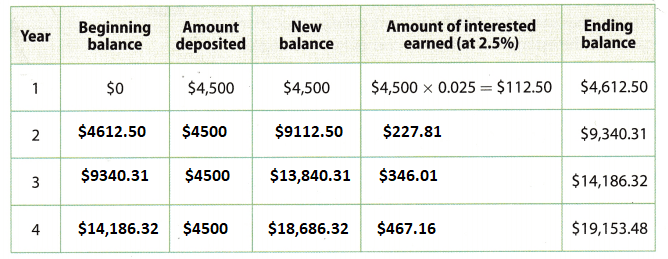Reflect

Question 5.
Does June have enough in her savings account to cover her first year at Texas A&M University-Kingsville without help from her father or a scholarship? What about with the scholarship?
As per Example 1, one year will cost an estimated $20,496 and she has only$19,153, hence she cannot cover her first year with her savings account alone.
The scholarship fees of $21,153 will be able to pay for her first year. Question 6. If June had been able to deposit$5,000 a year instead of $4,500, earning the same annual interest rate of 2.5%, would she have enough saved to pay for her first year? Answer: Given, June had deposited$5000 instead of $4500 for a year. Interest rate = 2.5% Formula for simple interest equal to SI = PTR/100 Here, P = actual money =$5000
T = time = 1 years.
R = interest rate = 2.5%
Simple interest for 1 years = $5000 × 1 × 2.5/100 =$125.
June fee for one year = $5738 Now he has =$5000 + $125 =$5125.
Therefore after the deposit of $5000 also he had less money than the college fee. Texas Go Math Grade 8 Lesson 16.4 Guided Practice Answer Key Ronan, a 19-year-old male from Texas, has been accepted at the University of Texas at Austin. If he attends the University of Texas, he plans to live at home with his mother, a single parent. His mother is a nurse who makes roughly$60,000 a year and pays roughly 13% in taxes annually. Ronan has never had a job. (Example 1, Explore Activity)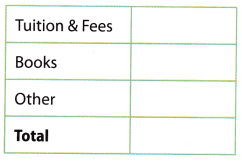Question 1.
Use the table and an online tool to estimate the cost of Ronan attending the University of Texas for 1 year.
The cost of Ronan attending the University of Texas for one-year Tuition and fees = $9794 The cost of books for one year =$904
The cost other for one year = $3752 The total cost of Ronan attending the university of texas for one year =$9794 + $904 +$3752 = $14,450. Question 2. Estimate the cost of Ronan getting a 4-year degree from the University of Texas. Answer: The total cost of Ronan attending the university of texas for one year =$14,450
The total cost of Ronan attending the university of texas for four years = $14,450 × 4 =$57,800

Question 3.
Ronan has been granted a scholarship for $1,500 per year. His mother has saved$21,000 for Ronan’s college education. Recalculate the estimated remaining cost of Ronan’s degree.
Given that,
Ronan has been granted a scholarship for one year = $1,500 For four years =$1500 × 4 = $6000. Mother saved for Ronan’s college education =$21,000.
The total money at Ronan = $6000 +$21000 = $27000 The total cost of Ronan attending the university of texas for four years =$57800.
Remaining cost of Ronan’s degree = $57800 –$27,000 = $30,800 Essential Question Check-In Question 4. What are some things to consider when estimating the cost of college? Answer: 1. Will you attend in/out state school? 2. Do you plan on living at home? 3. Do you have any savings or not to pay the fees? 4. Are you eligible for scholarships? Texas Go Math Grade 8 Lesson 16.4 Independent Practice Answer Key Question 5. At the beginning of each of the last two years, Laura put$4800 from her earnings as a part-time cashier during high school into a college savings account earning 1.2% interest compounded annually. Now she is applying for school and needs to know how much she has in her account. Complete the table to determine how much money Laura has saved.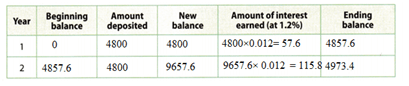Laura saved from her earnings as a part-time cashier during high school into a college savings account earning = $4800. Interest = 1.2% Question 6. At the beginning of each of the last three years, Lucas put$7000 from his earnings as a waiter into a college savings account that earned 1.5% interest compounded annually. Now he wants to attend community college for 2 years without taking out a loan. The cost of college will be about $18,000. Complete the table to determine whether Lucas has saved enough money to attend a community college.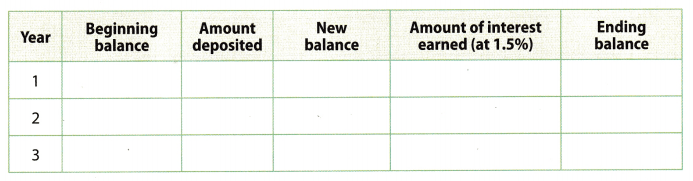Answer: Lucas saved from his earnings as a waiter into a college savings account that earned =$1800.
Interest = 1.5%Question 7.
Find a college grant online.
a. Grant Name:
Answer: College grants online are Federal Pell Grants.

b. Describe the application process.
Answer: To apply for Federal Pell Grants. First, you should fill out the FAFSA form and submit it. Then you will have to fill out the FAFSA form every year you are in school and demonstrate financial need to stay eligible for federal students.

c. How much money does the grant award?
Answer: The money for the grant award is $6995. Question 8. Find a college scholarship, a. Name of Scholarship: Answer: The name of the scholarship is the Tennessee HOPE scholarship. b. Describe the application process. Answer: • First login to the student’s login. • Filling in the scholarship application. • Upload all the documents related to education. • Submit the form to the respective educational institution. c. How much money does the scholarship award? Answer: The money award for the scholarship is$2000. It is not available for the summer semester.

H.O.T. Focus on Higher Order Thinking

Question 9.
Critical Thinking Having a savings plan is important even if you are not currently planning on attending college. Describe your savings plan, including stating a goal, how much you plan to save, and how you plan to save your money.